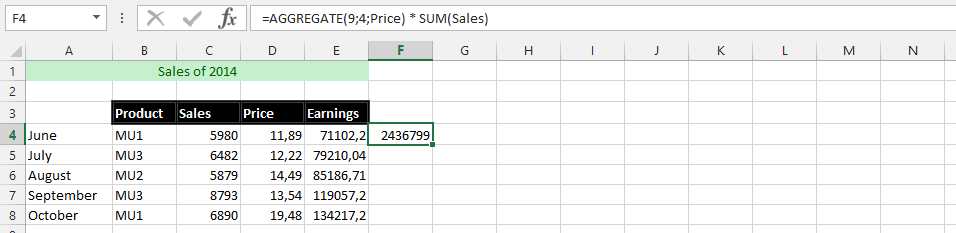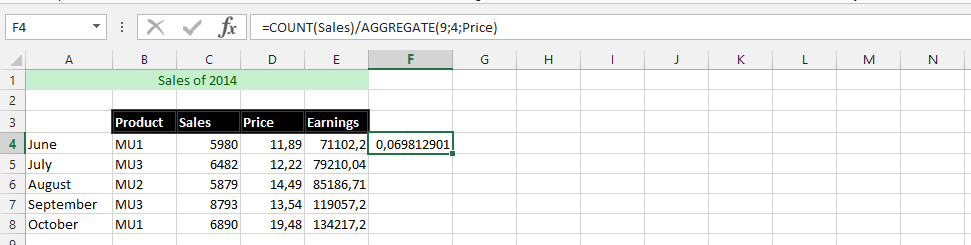#### How to Use Aggregate Function?

The aggregate function is a very useful function in Excel that can perform almost twenty different tasks with ease. It is a function that will return an aggregation in either a database or list. It has the ability to ignore certain areas that the user has chosen.

## Aggregate Function’s Arguments

The aggregate function has a syntax that works together in providing the values that it returns, which are in two different forms. One is the reference form, which has function numbers, options, and references that go from 1 to about 253 different ones. Another is the array form, where the function number is followed by options, the array, and finally the k.

Function number (function_num): This is a required part of the argument, and there are between 1 and 19 for the user to specify which function that he or she would like to use.

Options: This is another required argument, which is a numerical value that will determine which values are to be ignored in the evaluation range for functioning, which is between 0 and 7, omitted.

Reference 1 [ref1]: This is required and the first argument is a number for a function that will take different arguments of a number for which the user wishes to aggregate its value.

Reference 2+: [ref2]: An optional part of the arguments that contains a number and extends from 2 to 253 different arguments for the user to use.

## Aggregate Function’s Examples

### A Simple Formula

This is the example that clarifies that it is possible to use the aggregation formula to find the average of various prices we have set for the products over the months. We are going to use the aggregation formula to find the result.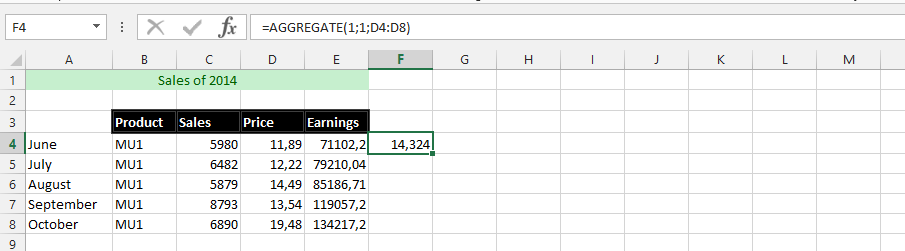### Aggregate Twice at the Same Time

The business has been going through some changes, and we needed to know what it looked like, so we could find the answers that we are looking for, and because we have changed the prices so much, we needed to know how much we have made. The formulas will make it possible to find out how much we have made in the business, which is informed by sales and all the dynamic changes we have made to the price setting of the same product.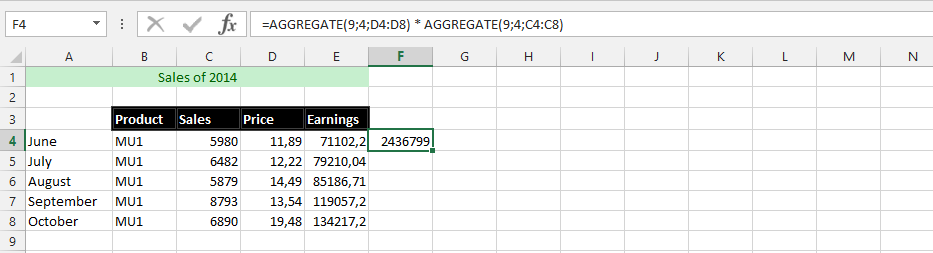### Using Aggregation to Solve a Mystery

#### Using Aggregation to Solve a Mystery Part 1

There is a little mystery that we are trying to solve. We need to find a simple resource that will explain the answers that we are looking for, which is simply about verifying that the earnings are truly the amount of money we have made in earnings. This is to clarify that we need to know the minimum amount of money that we will make if we sell all the products at the minimum price.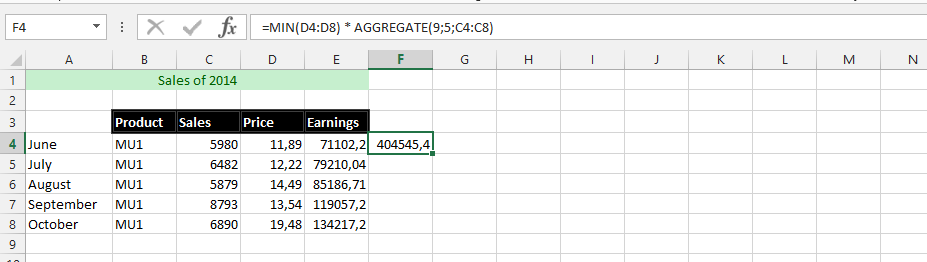#### Using Aggregation to Solve a Mystery Part 2

This example is about making it possible to know the maximum amount of money we make if we sell all the products at the highest price rate. This is where the formulas would be different from the information in the previous example.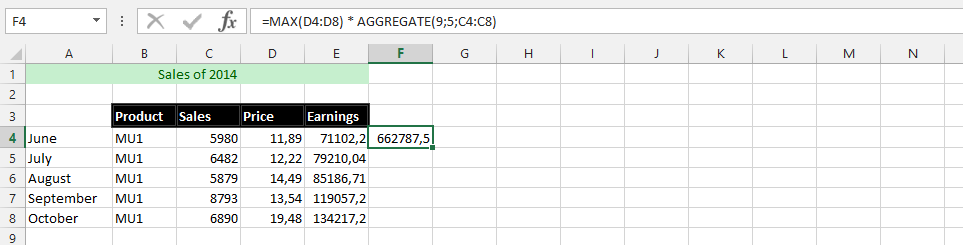#### Aggregation Solving a Mystery Part 3

This is about finding out how the average performance is with the aggregation formula, which means using the average formula with the aggregation formula, to find out how much we have made in average.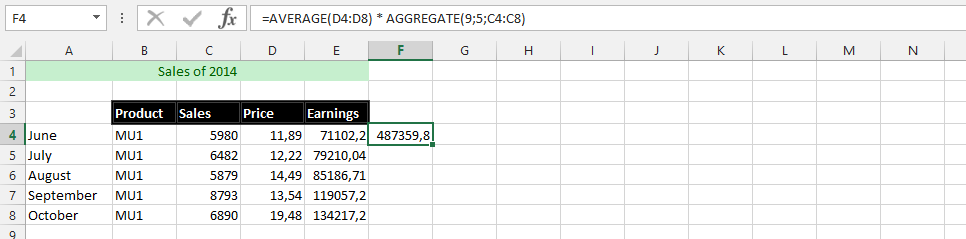### Creativity with Aggregation Formula

This example is going to provide the possibility of finding an appropriate and correct answer, which makes it possible to know how the average performance would affect the whole performance of the business. This is where it is necessary to know what is best for the company, because it makes it easy to make different assessments.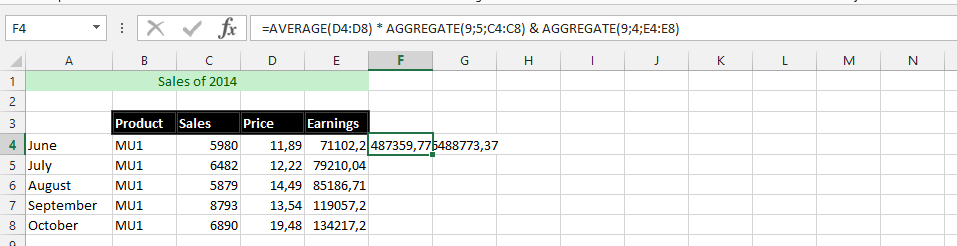### VLOOKUP Usage with AGGREGATION

This example uses the combination of Vlookup and the aggregation formula together to increase the success of finding a specific answer. This is why we are going to use both formulas in one single formula to find the answer.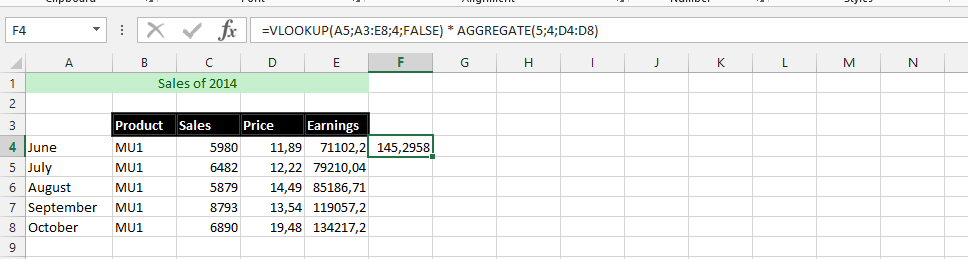### Two Aggregate to Divide

We are using two aggregation formulas, but this time there is a divide between them. We are going to use this to find answers with the information, but we have labeled all the data with texts, which is what we are going to use to find the answers we are looking for, which the picture below provides.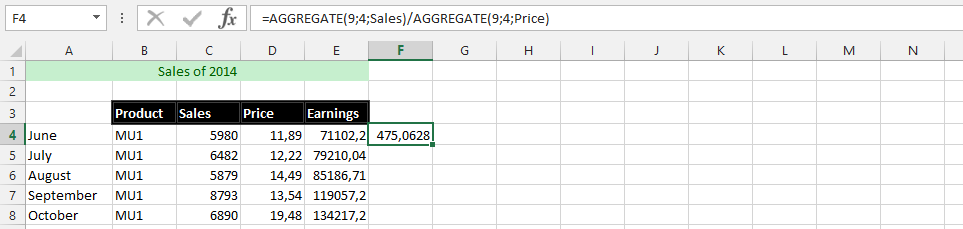### SUM and AGGREGATION

This is an example where we are using both the sum and aggregation to find the total value that we will be using when the whole information is very clear. We want to use both the sum and aggregation functions to get an idea of how much we could make from the information.